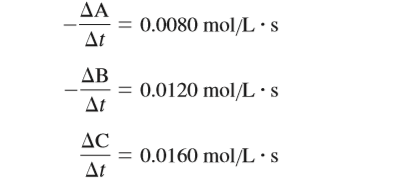# Problem: Consider the general reaction aA + bB → cC and the following average rate data over some time period Δt:Determine a set of possible coefficients to balance this general reaction.

###### FREE Expert Solution

We are asked to determine a set of possible coefficients to balance the given general reaction:

aA + bB → cC

Given:

82% (326 ratings)###### Problem Details

Consider the general reaction

aA + bB → cC

and the following average rate data over some time period Δt:Determine a set of possible coefficients to balance this general reaction.